# Selina Solutions Concise Maths Class 10 Chapter 22 Heights and Distances Exercise 22(A)

The exercise problems give students a basic understanding of the angle of elevation and depression. Students willing to strengthen their fundamentals of ICSE Class 10 Mathematics can make use of the Selina Solutions for Class 10 Maths. BYJU’S has prepared all the solutions, keeping in mind the needs of all students. The Concise Selina Solutions for Class 10 Maths Chapter 22 Heights and Distances Exercise 22(A) PDF can be downloaded from the link given below.

## Selina Solutions Concise Maths Class 10 Chapter 22 Heights and Distances Exercise 22(A) Download PDF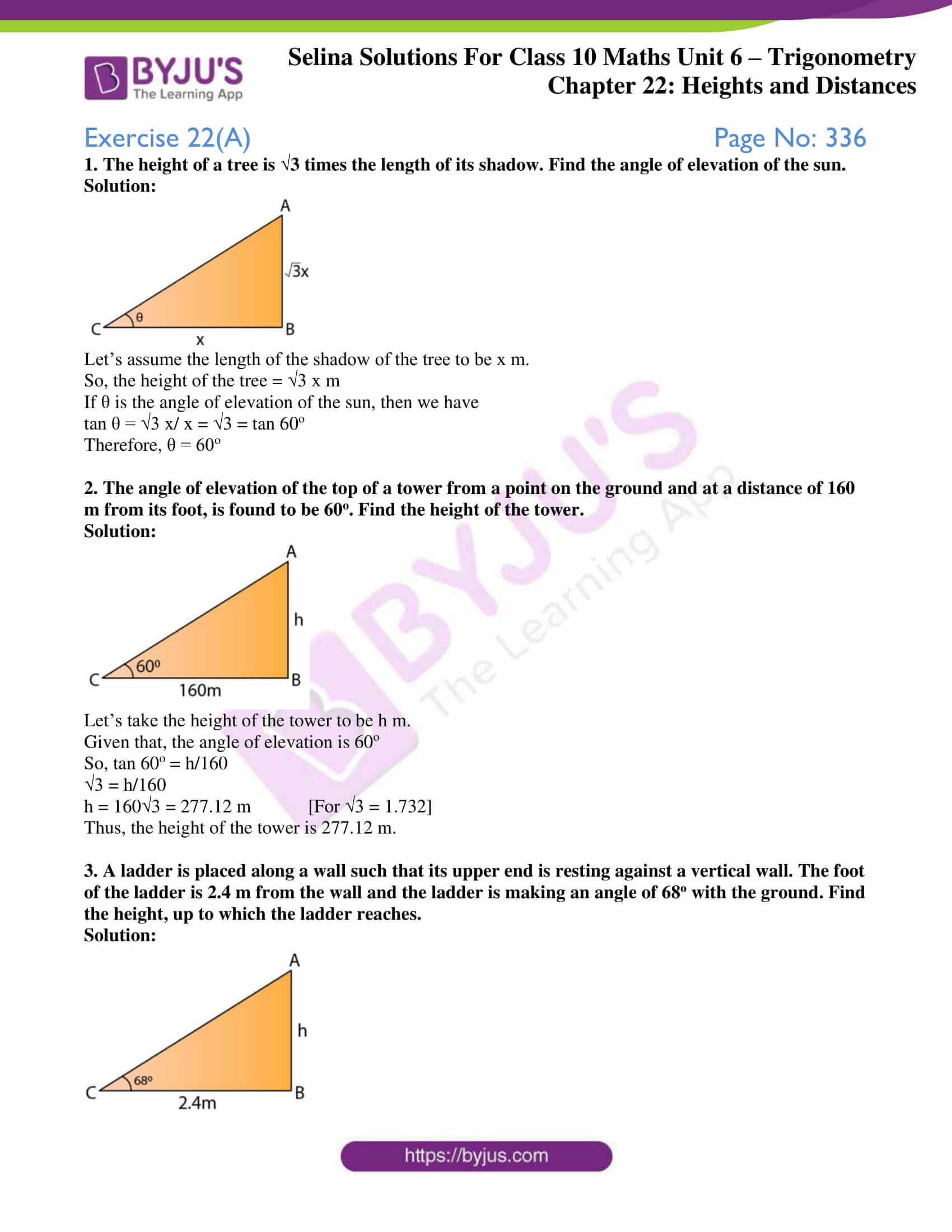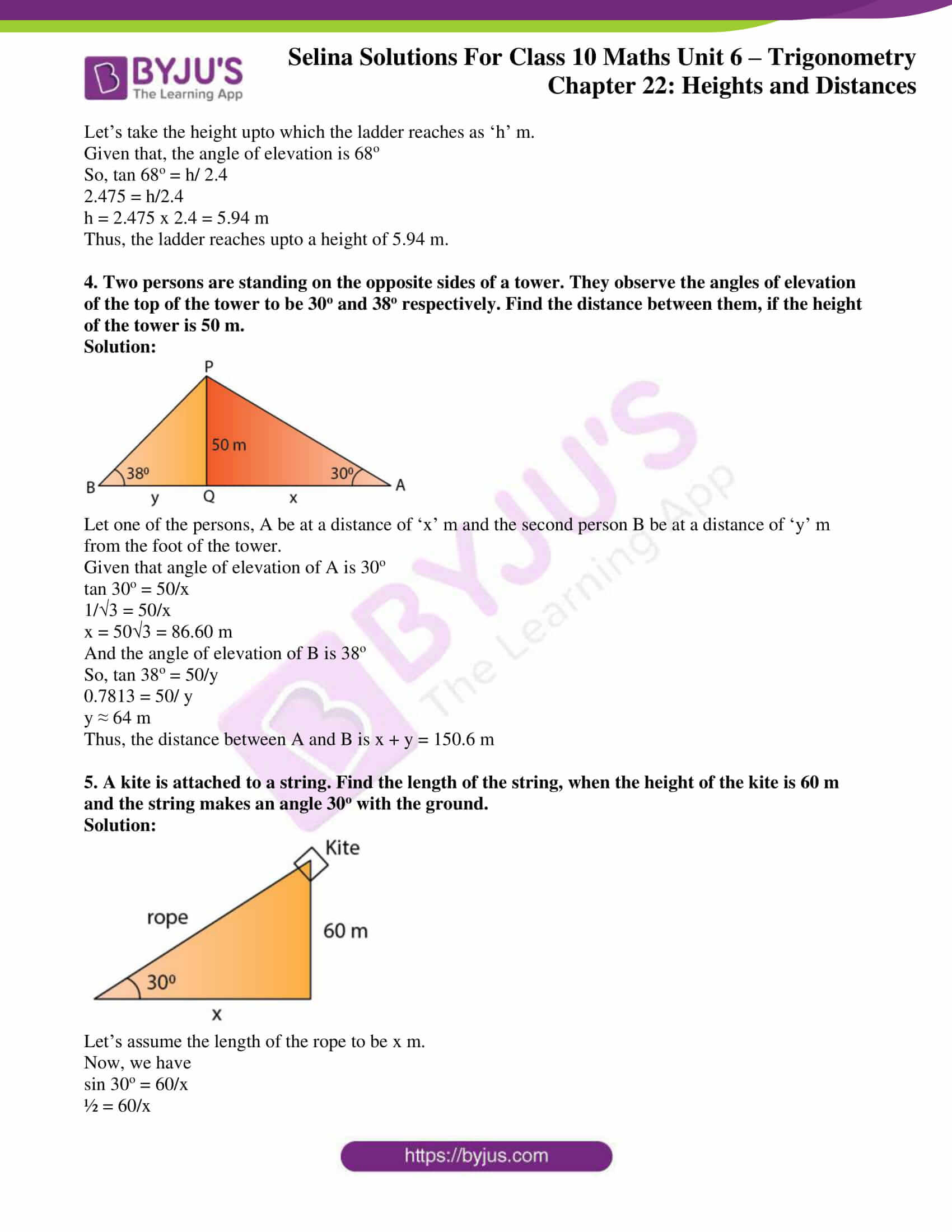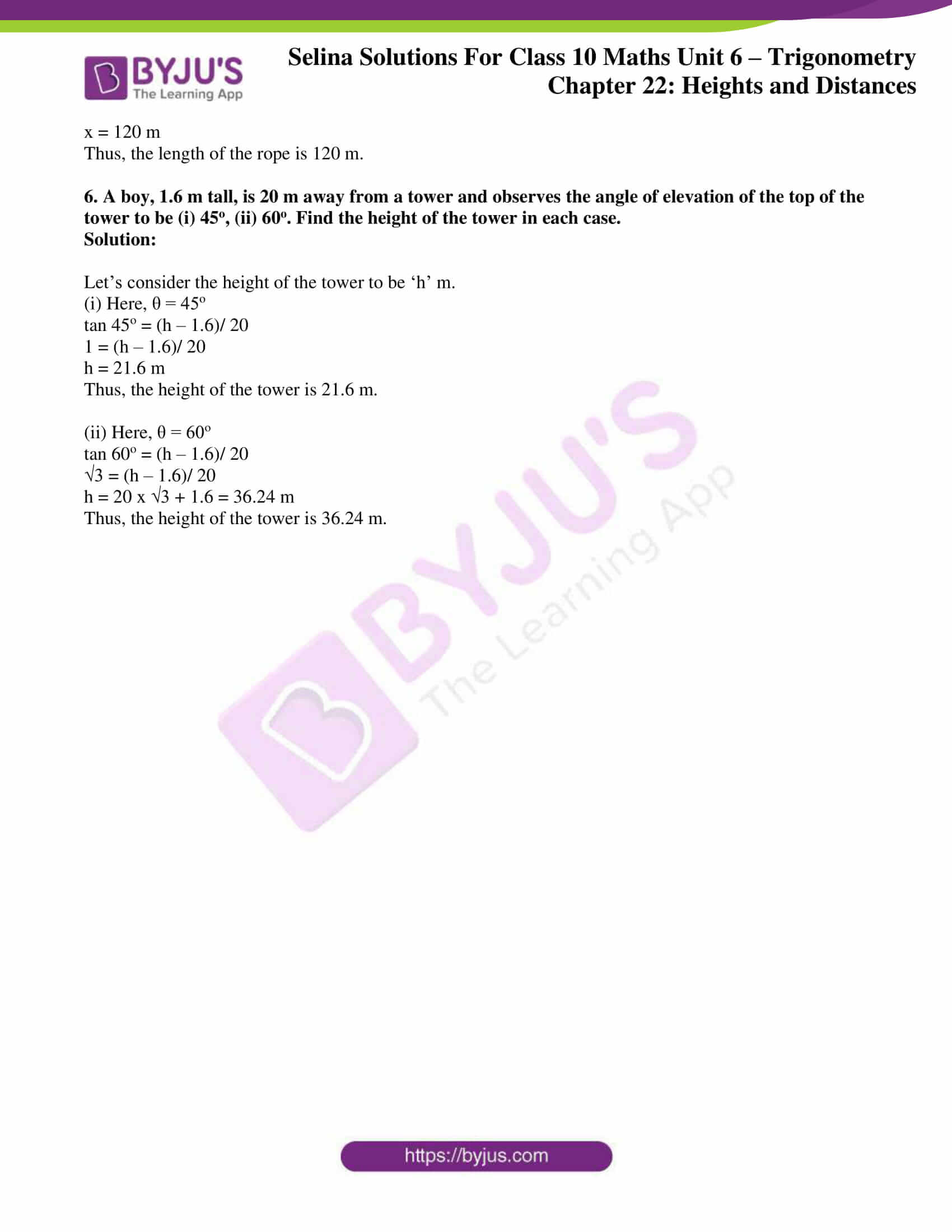### Access Selina Solutions Concise Maths Class 10 Chapter 22 Heights and Distances Exercise 22(A)

1. The height of a tree is √3 times the length of its shadow. Find the angle of elevation of the sun.

Solution: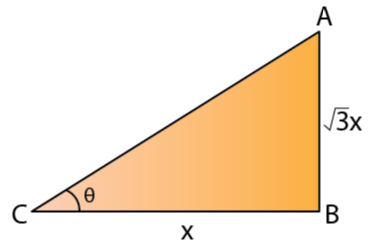Let’s assume the length of the shadow of the tree to be x m.

So, the height of the tree = √3 x m

If θ is the angle of elevation of the sun, then we have

tan θ = √3 x/ x = √3 = tan 60o

Therefore, θ = 60o

2. The angle of elevation of the top of a tower from a point on the ground and at a distance of 160 m from its foot, is found to be 60o. Find the height of the tower.

Solution: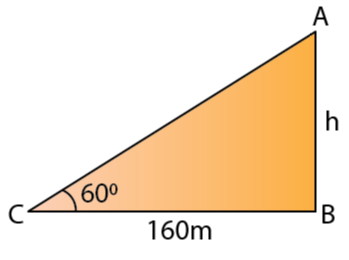Let’s take the height of the tower to be h m.

Given that, the angle of elevation is 60o

So, tan 60o = h/160

√3 = h/160

h = 160√3 = 277.12 m [For √3 = 1.732]

Thus, the height of the tower is 277.12 m.

3. A ladder is placed along a wall such that its upper end is resting against a vertical wall. The foot of the ladder is 2.4 m from the wall and the ladder is making an angle of 68o with the ground. Find the height, up to which the ladder reaches.

Solution: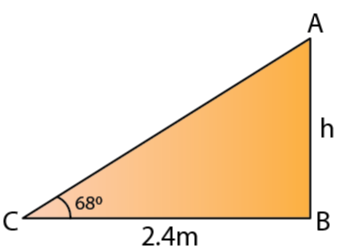Let’s take the height upto which the ladder reaches as ‘h’ m.

Given that, the angle of elevation is 68o

So, tan 68o = h/ 2.4

2.475 = h/2.4

h = 2.475 x 2.4 = 5.94 m

Thus, the ladder reaches upto a height of 5.94 m.

4. Two persons are standing on the opposite sides of a tower. They observe the angles of elevation of the top of the tower to be 30o and 38o respectively. Find the distance between them, if the height of the tower is 50 m.

Solution: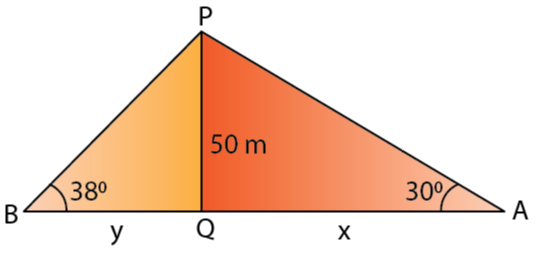Let one of the persons, A be at a distance of ‘x’ m and the second person B be at a distance of ‘y’ m from the foot of the tower.

Given that angle of elevation of A is 30o

tan 30o = 50/x

1/√3 = 50/x

x = 50√3 = 86.60 m

And the angle of elevation of B is 38o

So, tan 38o = 50/y

0.7813 = 50/ y

y ≈ 64 m

Thus, the distance between A and B is x + y = 150.6 m

5. A kite is attached to a string. Find the length of the string, when the height of the kite is 60 m and the string makes an angle 30o with the ground.

Solution: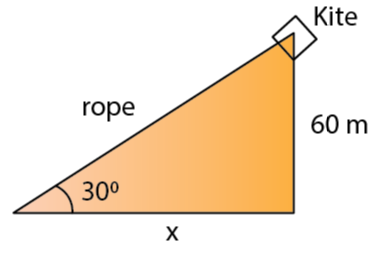Let’s assume the length of the rope to be x m.

Now, we have

sin 30o = 60/x

½ = 60/x

x = 120 m

Thus, the length of the rope is 120 m.

6. A boy, 1.6 m tall, is 20 m away from a tower and observes the angle of elevation of the top of the tower to be (i) 45o, (ii) 60o. Find the height of the tower in each case.

Solution:

Let’s consider the height of the tower to be ‘h’ m.

(i) Here, θ = 45o

tan 45o = (h – 1.6)/ 20

1 = (h – 1.6)/ 20

h = 21.6 m

Thus, the height of the tower is 21.6 m.

(ii) Here, θ = 60o

tan 60o = (h – 1.6)/ 20

√3 = (h – 1.6)/ 20

h = 20 x √3 + 1.6 = 36.24 m

Thus, the height of the tower is 36.24 m.